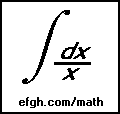# A Conjecture on Collections of Subsets

#### September 3, 2002

NOTE: A search of the Internet finally revealed that this conjecture is true and is known as Sperner's Theorem.

Here's a problem in combinatorics that has bugged me for many years. Perhaps a combinatoricist can solve it at once. I couldn't.

Consider a finite set S of N objects. A collection without inclusion is a collection C of subsets of S such that no subset in the collection is a proper subset of any other subset in the collection.

One kind of collection without inclusion is the collection of all single-element subsets of S. Another is the collection of all two-element subsets. However, there are many, many others.

Here is the conjecture: The collection of N/2-element subsets contains at least as many subsets as any other collection without inclusion. Here N/2 is rounded either up or down if N is odd. It doesn't make any difference, because the size of the collection is the same in either case.

Brute-force testing by computer can verify this conjecture only for very small values of N. The number of subsets is P = 2N, and the number of collections of subsets is 2P, which gets very large very fast!

The application that gave rise to this conjecture is probably obsolete by now, but here it is. There used to be something called a PROM (programmable read-only memory). It was manufactured containing all zeros. By using a special machine that selectively burned out internal connections, the user could change any zero into a one, but not vice-versa. That was called "burning" the PROM.

Now suppose each word of the PROM contains N bits. If you code the information so exactly N/2 bits of each word are changed to ones, the information cannot be changed and remain valid. If there is a collection without inclusion larger than the collection of all N/2-element subsets, there is a more efficient way to do this.